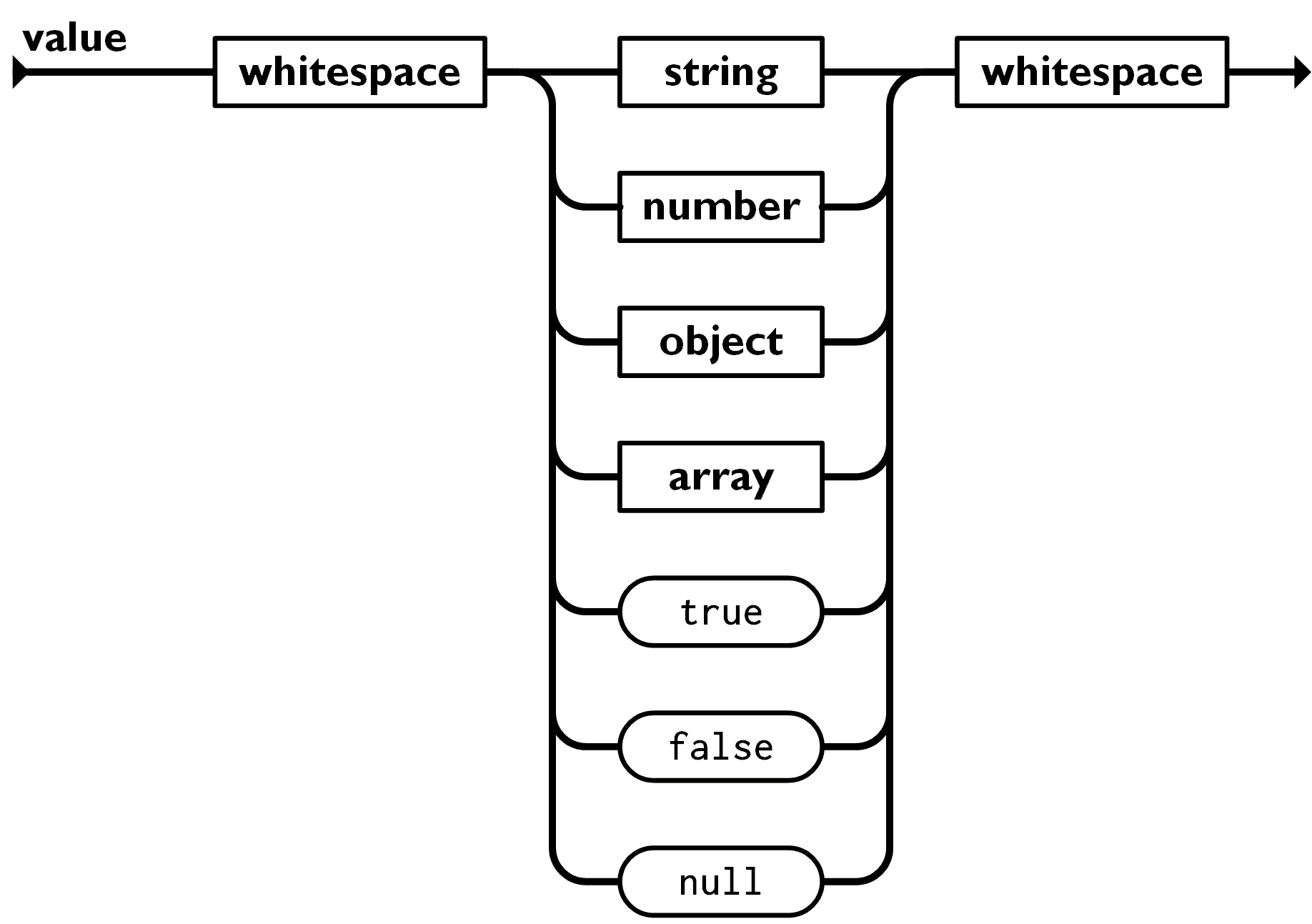# 精读《手写 JSON Parser》

## 1 引言

JSON.parse 是浏览器内置的 API，但如果面试官让你实现一个怎么办？好在有人已经帮忙做了这件事，本周我们一起精读这篇 JSON Parser with Javascript 文章吧，再温习一遍大学时编译原理相关知识。

## 2 概述 & 精读### 实现 Parser

function fakeParseJSON(str) {
let i = 0;
// TODO
}

i 表示访问字符的下标，当 i 走到字符串结尾表示遍历结束。

function fakeParseJSON(str) {
let i = 0;
function parseObject() {
if (str[i] === '{') {
i++;
skipWhitespace();

// if it is not '}',
// we take the path of string -> whitespace -> ':' -> value -> ...
while (str[i] !== '}') {
const key = parseString();
skipWhitespace();
eatColon();
const value = parseValue();
}
}
}
}

function fakeParseJSON(str) {
let i = 0;
function parseObject() {
if (str[i] === '{') {
i++;
skipWhitespace();

let initial = true;
// if it is not '}',
// we take the path of string -> whitespace -> ':' -> value -> ...
while (str[i] !== '}') {
if (!initial) {
eatComma();
skipWhitespace();
}
const key = parseString();
skipWhitespace();
eatColon();
const value = parseValue();
initial = false;
}
// move to the next character of '}'
i++;
}
}
}

function fakeParseJSON(str) {
// ...
function eatComma() {
if (str[i] !== ',') {
throw new Error('Expected ",".');
}
i++;
}

function eatColon() {
if (str[i] !== ':') {
throw new Error('Expected ":".');
}
i++;
}
}

function fakeParseJSON(str) {
let i = 0;
function parseObject() {
if (str[i] === '{') {
i++;
skipWhitespace();

const result = {};

let initial = true;
// if it is not '}',
// we take the path of string -> whitespace -> ':' -> value -> ...
while (str[i] !== '}') {
if (!initial) {
eatComma();
skipWhitespace();
}
const key = parseString();
skipWhitespace();
eatColon();
const value = parseValue();
result[key] = value;
initial = false;
}
// move to the next character of '}'
i++;

return result;
}
}
}function fakeParseJSON(str) {
// ...
function parseArray() {
if (str[i] === '[') {
i++;
skipWhitespace();

const result = [];
let initial = true;
while (str[i] !== ']') {
if (!initial) {
eatComma();
}
const value = parseValue();
result.push(value);
initial = false;
}
// move to the next character of ']'
i++;
return result;
}
}
}function fakeParseJSON(str) {
// ...
function parseValue() {
skipWhitespace();
const value =
parseString() ??
parseNumber() ??
parseObject() ??
parseArray() ??
parseKeyword('true', true) ??
parseKeyword('false', false) ??
parseKeyword('null', null);
skipWhitespace();
return value;
}
}

function fakeParseJSON(str) {
// ...
function parseKeyword(name, value) {
if (str.slice(i, i + name.length) === name) {
i += name.length;
return value;
}
}
}

### 处理异常输入

1. 非法字符。
2. 非正常结尾。

Unexpected token "a"

// show
{ "b"a
^
JSON_ERROR_001 Unexpected token "a".
Expecting a ":" over here, eg:
{ "b": "bar" }
^
You can learn more about valid JSON string in http://goo.gl/xxxxx

## 3 总结

syntax-parser 这个零依赖的通用语法解析库就是根据上述文章一步一步完成的，看完了上面文章，就彻底理解了这个库的源码。